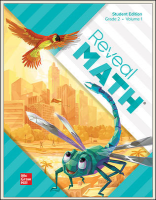1 Unit 1 Math Is... Lesson 1-1: Math Is Mine Lesson 1-2: Math Is Exploring and Thinking Also consider: Lesson 1-1: Math Is Mine Lesson 1-2: Math Is Exploring and Thinking Also consider: Lesson 1-3: Math Is In My World Lesson 1-4: Math Is Explaining and Sharing Also consider: Lesson 1-3: Math Is In My World Lesson 1-4: Math Is Explaining and Sharing Also consider: Lesson 1-5: Math Is Finding Patterns Lesson 1-6: Math Is Ours Lesson 1-5: Math Is Finding Patterns Lesson 1-6: Math Is Ours
 2 Unit 2 Place Value to 1,000 Lesson 2-1:  Understand Hundreds Lesson 2-1:  Understand Hundreds Lesson 2-2:  Understand 3-Digit Numbers Also consider: Lesson 2-2:  Understand 3-Digit Numbers Also consider: Lesson 2-3:  Read and Write Numbers to 1,000 Lesson 2-3:  Read and Write Numbers to 1,000 Lesson 2-4:  Decompose 3-Digit Numbers Lesson 2-4:  Decompose 3-Digit Numbers Lesson 2-5:  Compare 3-Digit Numbers Also consider: Lesson 2-5:  Compare 3-Digit Numbers Also consider:
 3 Unit 3 Patterns within Numbers Lesson 3-1:  Counting Patterns Lesson 3-1:  Counting Patterns Lesson 3-2:  Patterns When Skip Counting by 5s Lesson 3-2:  Patterns When Skip Counting by 5s Lesson 3-3:  Patterns When Skip Counting by 10s and 100s Also consider: Lesson 3-3:  Patterns When Skip Counting by 10s and 100s Also consider: Lesson 3-4:  Understanding Even and Odd Numbers Lesson 3-4:  Understanding Even and Odd Numbers Lesson 3-5:  Addition Patterns Lesson 3-5:  Addition Patterns Lesson 3-6:  Patterns with Arrays Lesson 3-6:  Patterns with Arrays Lesson 3-7:  Use Arrays to Add Lesson 3-7:  Use Arrays to Add
 4 Unit 4 Meanings of Addition and Subtraction Lesson 4-1:  Represent and Solve Add To Problems Lesson 4-1:  Represent and Solve Add To Problems Lesson 4-2:  Represent and Solve Take From Problems Lesson 4-2:  Represent and Solve Take From Problems Lesson 4-3:  Solve Two-Step Add To and Take From Problems Lesson 4-3:  Solve Two-Step Add To and Take From Problems Lesson 4-4:  Represent and Solve Put Together Problems Lesson 4-4:  Represent and Solve Put Together Problems Lesson 4-5:  Represent and Solve Take Apart Problems Lesson 4-5:  Represent and Solve Take Apart Problems Lesson 4-6:  Solve Two-Step Put Together and Take Apart Problems Lesson 4-6:  Solve Two-Step Put Together and Take Apart Problems Lesson 4-7:  Represent and Solve Compare Problems Lesson 4-7:  Represent and Solve Compare Problems Lesson 4-8:  Represent and Solve More Compare Problems Lesson 4-8:  Represent and Solve More Compare Problems Lesson 4-9:  Solve Two-Step Problems with Comparison Lesson 4-9:  Solve Two-Step Problems with Comparison Lesson 4-10:  Solve Two-Step Problems Using Addition and Subtraction Lesson 4-10:  Solve Two-Step Problems Using Addition and Subtraction